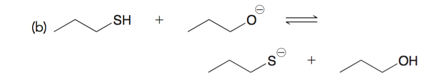# Problem: For each reaction below, draw the mechanism (curved arrows) and then predict which side of the reaction is favored under equilibrium conditions.

###### FREE Expert Solution
90% (449 ratings)
###### Problem Details

For each reaction below, draw the mechanism (curved arrows) and then predict which side of the reaction is favored under equilibrium conditions.What scientific concept do you need to know in order to solve this problem?

Our tutors have indicated that to solve this problem you will need to apply the Acid Base Equilibrium concept. You can view video lessons to learn Acid Base Equilibrium. Or if you need more Acid Base Equilibrium practice, you can also practice Acid Base Equilibrium practice problems.

What is the difficulty of this problem?

Our tutors rated the difficulty ofFor each reaction below, draw the mechanism (curved arrows) ...as high difficulty.

How long does this problem take to solve?

Our expert Organic tutor, Jonathan took 1 minute and 57 seconds to solve this problem. You can follow their steps in the video explanation above.

What professor is this problem relevant for?

Based on our data, we think this problem is relevant for Professor Kahnamoui's class at WWU.

What textbook is this problem found in?

Our data indicates that this problem or a close variation was asked in Organic Chemistry - Klein 1st Edition. You can also practice Organic Chemistry - Klein 1st Edition practice problems.